Support

Apps

•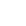My Wallet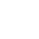My Order
•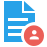My Profile
•My Connections
•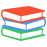My Books
•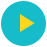My Videos
•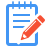My Tests
•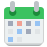My Calender
•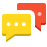My Messages
•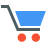My Shopping Cart
•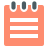My Orders
•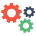Account Settings
•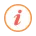Help

# Book Details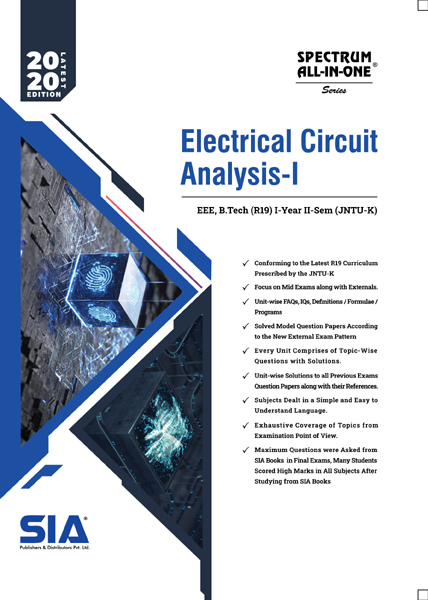# Electrical Circuits Analysis-I (JNTU-K)

 Course Code : B.Tech I-II (EEE) R19 Author : SIA PUBLISHERS University : JNTU Kakinada Regulation : 2020 Categories : Engineering Format :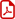PDF (DRM Protected) Type : eBook

Rs.189 Rs.104 Rs.45% off

Description :

SYLLABUS

UNIT-I

INTRODUCTION TO ELECTRICAL CIRCUITS Basic concepts of passive elements of R, L, C and their V-I relations, Sources (dependent and independent) – Kirchhoff’s laws, Network reduction techniques (Series, Parallel, Series-parallel, Star-to-delta and delta-tostar transformation). Source transformation technique, Nodal analysis and mesh analysis to D.C networks with dependent and independent voltage and current sources.

UNIT-II

MAGNETIC CIRCUITS Basic definition of MMF, Flux and reluctance, Analogy between electrical and magnetic circuits, Faraday’s laws of electromagnetic induction – Concept of self and mutual inductance, Dot convention-coefficient of coupling and composite magnetic circuit, Analysis of series and parallel magnetic circuits.

UNIT-III

SINGLE PHASE A.C SYSTEMS Periodic waveforms (determination of r.m.s, average value and form factor), Concept of phase angle and phase difference – Waveforms and phasor diagrams for lagging, Leading networks, Complex and polar forms of representations. Steady state analysis of R, L and C circuits, Power factor and its significance, Real reactive power and apparent power, Waveform of instantaneous power and complex power.

UNIT-IV

ANALYSIS OF A.C NETWORKS Extension of node and mesh analysis of A.C networks, Series and parallel resonance, Selectivety, bandwidth and Quality factor, Introduction to locus diagram.

UNIT-V

NETWORK THEOREMS (D.C AND A.C EXCITATIONS) Superposition theorem, Thevenin’s theorem, Norton’s theorem, Maximum power transfer theorem, Reciprocity theorem, Millman’s theorem and compensation theorem

Note : Electrical Circuits Analysis-I (JNTU-K) B.Tech I-II (EEE) R19

##### Publisher Detail:

Publisher Name: SIA Publishers and Distributors (P) Ltd.

Contact Email Id: msultan@siaedugroup.com